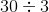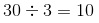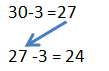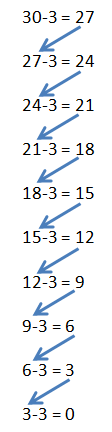# How to Divide by 3

Instructor: Jennifer Zaur

Jennifer has taught at both the elementary and university level. She holds a master’s degree in curriculum and instruction.

In this lesson, you'll see several different strategies you can use to help you successfully learn how to divide by 3. Learn about making groups, repeated subtraction, and skip counting.

## What Does it Mean to Divide by 3?

Have you ever had two friends over to your house and you decided that you wanted to share a bag of candy evenly with them? In order to make sure that everyone gets the same number of pieces of candy, you have to take all of the candies and break them up into three groups. This is dividing by 3.

When you divide by 3, you take a number and break it up into three groups. Now, imagine that when you opened the bag of candy you wanted to share with your friends, it had 30 pieces of candy inside. In order to figure out how many pieces each person gets, you would have to solve the problem:So, just how to do you do this? Let's find out.

## Making Groups

One way to solve the problem is by making groups. When you make groups, you take the total number, and break it up into three groups. Let's practice making groups by solving the problem:To do this, start by drawing three circles to represent the three groups you are making.Next, you will add one candy to the first group, one candy to the second group, and one candy to the third group.Keep adding one candy at a time to each of your three groups, one after the other and in the same order, until you have no more candies to add. Once you have added each of your candies to a group, count the number of candies in each group. The number of candies in each group is the answer to your division problem. So, since there are 10 candies in each group:## Repeated Subtraction

Another way that you can divide by 3 is to use repeated subtraction. To use repeated subtraction to solve the problem, you would turn the division problem into a subtraction problem. So, the math problem would become 30 - 3. Then, you solve the subtraction problem:

30 - 3 = 27

After you have solved the subtraction problem, you move the answer to the subtraction problem so that it becomes the first number in a new subtraction problem - where you subtract 3 again - like this:Continue following this process until you reach a subtraction equation where you cannot take away 3 anymore.To unlock this lesson you must be a Study.com Member.

### Register to view this lesson

Are you a student or a teacher?

Back

### Resources created by teachers for teachers

Over 30,000 video lessons & teaching resources‐all in one place.Video lessonsQuizzes & WorksheetsClassroom IntegrationLesson Plans

I would definitely recommend Study.com to my colleagues. It’s like a teacher waved a magic wand and did the work for me. I feel like it’s a lifeline.

Jennifer B.
TeacherCreate an account to start this course today
Used by over 30 million students worldwide